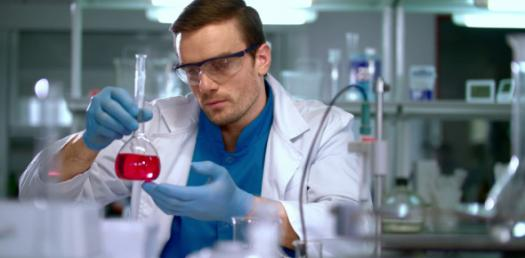# Rutherford's Experiment

10 Questions | Total Attempts: 488SettingsRutherford’s Gold Foil Experiment is what proved the existence of a small massive centre to atoms, which would later come to be known as the nucleus of the atom. What do you know about the experiment? Let’s have a look!

Related Topics
• 1.
Step 1. Open the Plum Pudding atom.  The blue dots represent which of the following?
• A.

Proton

• B.

Neutron

• C.

Electron

• D.

Photon

• E.

Positively charged "pudding"

• 2.
The red dots represent which of the following
• A.

Proton

• B.

Neutron

• C.

Electron

• D.

Photon

• E.

Positively charged "pudding"

• 3.
The white dots represent which of the following
• A.

Proton

• B.

Neutron

• C.

Electron

• D.

Photon

• E.

Positively charge "pudding"

• 4.
The red "blob" represents which of the following
• A.

Protons

• B.

Neutrons

• C.

Electrons

• D.

Positively charged "pudding"

• 5.
The "Ray gun" fires alpha particles.  What are alpha particles made of
• A.

2 protons 2 neutrons

• B.

2 protons 2 electrons

• C.

4 protons

• D.

4 neutrons

• E.

4 little blobs of positively charges "pudding"

• 6.
As the alpha particle passes through the "pudding" which of the following best describes the path of the alpha particle.
• A.

The particle is deflected back to the gun

• B.

The particles are pulled towards the center

• C.

The particles are slightly (if at all) deflected

• 7.
Thomson predicted that alpha particle would be on be slightly deflected as it passed through the sheet of gold foil because he believed that the positive charge was spread out over the volume of the atom like a pudding
• A.

True

• B.

False

• 8.
Step 2. Now open the tab titled "Rutherford's Atom".  Referring to only the electron what is the major difference between the two models
• A.

No major difference

• B.

The electron are stationary in the Rutherford model but not in Thomson model

• C.

The electron is orbiting in the the nucleus in the Thomson model but not the Rutherford model

• D.

The electron is orbiting in the the nucleus in the Rutherford model but not the Thomson model

• 9.
Referring to only the protons and neutrons what is the major difference between the two models
• A.

No major differences

• B.

The Rutherford model has the protons and neutrons located in the center of the atom, in the nucleus, but not the Thomson model

• C.

The Thomson model has the protons and neutrons located in the center of the atom, in the nucleus, but not the Rutherford model

• 10.
Of the two models which is correct?
• A.

Both

• B.

Neither, but the Rutherford model is closer to the excepted model

• C.

Neither, but the Thomson model is closer to the excepted model

• D.

Neither model is close to being correct